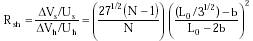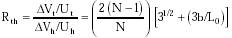Nanomedicine, Volume I: Basic Capabilities

Robert A. Freitas Jr., Nanomedicine, Volume I: Basic Capabilities, Landes Bioscience, Georgetown, TX, 1999

5.2.4.2 Tiling Deforming Surfaces

Most physiological surfaces are subject to periodic deformation, or stretching, along one or both dimensions. Consider the problem of tiling a surface that is periodically deforming in one direction only. A good example in human physiology is the large elastic arteries which circumferentially distend and contract as they absorb ~50% of the stroke volume of each ventricular ejection. Specifically, diameter oscillations in the pulmonary artery and aorta typically range from 9%-12%, averaging 11% for men and women under 35 years of age and 6.5% for people over 65.521 By contrast, vessel lengths display almost no variation at all.361,521

In order to tile such a monoaxially deforming surface, individual nanorobots must have the ability to expand in one dimension only, using their inflatable bumpers. The nanodevice shape which requires the smallest bumpers (i.e., minimum volume) to accommodate a given linear expansion is the most space-usage efficient and is probably preferred. Assuming continuous perimeter bumpers, compare a series of N square, hexagonal, or triangular prismatic nanorobots with equal sides of length s, height h, and fully contracted bumpers of minimum depth b (Fig. 5.3). The center-to-center linear distance between adjacent square units is L0 = s + 2b; between adjacent hexagonal units along the stretch axis, L0 = 3s/2 + 3b/31/2; and between adjacent triangular units along the stretch axis, L0 = s + 31/2 b.

Now assume the center-to-center distance increases by DL along the stretch axis. The increase in bumper volume for the squares is DVs = h DL L0 (N-1)/N per square. (For closed circuits such as complete circular rings of tiles, the (N-1)/N factor drops out.) The useful interior nanorobot volume (in which nonbumper machinery may be present) is Us = h s2 = h (L0 - 2b)2. For the hexagons, DVh = h DL L0 31/2 / 3 per hexagon, and Uh = h s2 271/2 / 2 where s = 2 ((L0/31/2) - b) / 31/2. For the triangles, DVt = (31/2 / 2) h DL (L0 + 31/2 b) (N-1)/N per triangle, and Ut = (31/2 / 4) h s2 where s = L0 - 31/2 b.

We may now compare the relative volumetric cost of square (Rsh) and triangular (Rth) device bumpers to hexagonal device bumpers using{Eqn. 5.1}{Eqn. 5.2}

For any choice of (b/L0) > 0, then Rsh > 1 for all N > 2 and Rth > 1 for all N > 0. That is, bumpers on square or triangular devices inevitably require a larger percentage change in nanorobot volume to effect a given linear adjustment, hence the hexagonal prism is always the more space-efficient shape for tasks requiring monoaxial deformations. (Squares are more efficient than triangles except for 0.43 L0 < b < 0.52 L0.)

Similar results follow from an analysis of tiling a surface that is simultaneously deforming in both dimensions. Most moving biological surfaces stretch along both axes at once, often at different rates and phases. Examples include the skin (which can stretch up to 100% before permanent damage is observed521), the abdominal diaphragm, cardiac chamber walls (papillary muscles thicken while shortening 7-20% during an isometric contraction362), alimentary surfaces, and various bladders, glands and sacs. Tendons typically stretch 5% (up to 10% in the human psoas tendon521) while contracting slightly in width. Apparently hexagonal prisms are also the most efficient shape for tessellating nanorobots aggregating on biaxially deforming surfaces; the intuitive rationale is that the most efficient shape is closest to a circle, with minimal perimeter length enclosing maximal area. In nature, epidermal squamous cells are arranged in hexagonal columns and onion skin cells also form neat arrays of hexagons. Hexagonal territorial partitioning is common among surface-feeding bird and fish species.2029,2030

Last updated on 17 February 2003